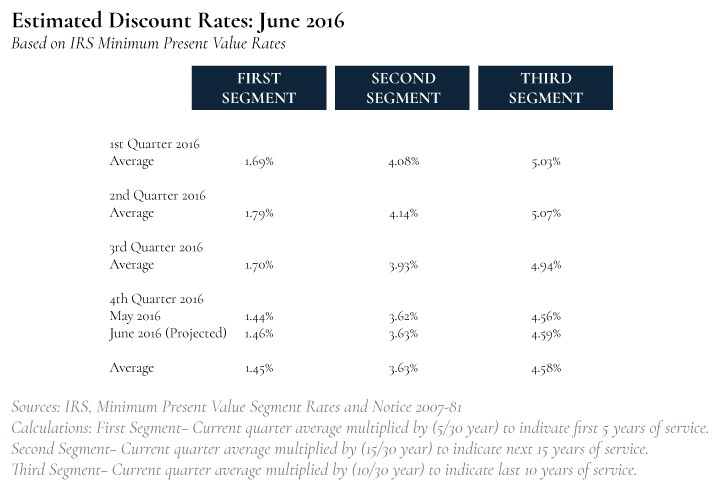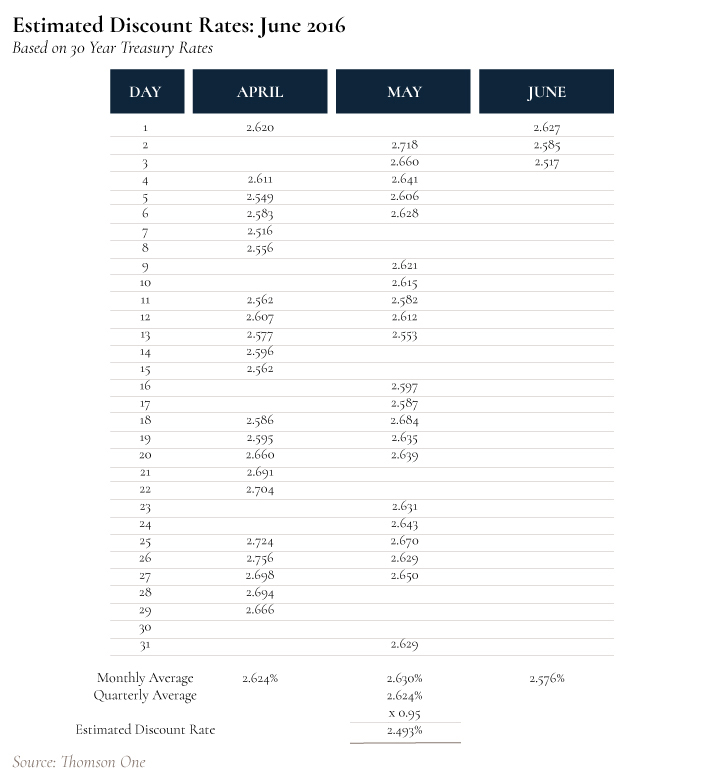# Estimated Discount Rates: June 2016

Scroll to Top

Based on 30 Year Treasury Rates

There are two age groups that this describes; the grandfathered group and anyone else. The grandfathered group gets their discount rate by taking 95% of the daily average of the 30-year Treasury bonds.

We know that this group’s discount rate for the 3rd quarter is 2.5% based on where rates have been so far for this quarter and we also know that the discount rate for fourth quarter will be 2.5% as well.

For the category of anyone else, we have to use a segmented blend of corporate bond rates, short, intermediate and long-term bonds. We have seen the spread between corporate bonds and treasury bonds narrowing, and trending downwards towards year-end.Estimated Discount Rates: June 2016

Based on IRS Minimum Present Value RatesSource(s):
2. IRS: Minimum Present Value Segment Rates
3. IRS: Notice 2007-81
4. Calculations – First Segment: Current quarter average multiplied by (5/30 year) to indicate first 5 years of service. Second Segment: Current quarter average multiplied by (15/30 year) to indicate next 15 years of service. Third segment: Current quarter average multiplied by (10/30 year) to indicate last 10 years of service.

2. IRS Minimum Present Value Segment Rates:

The first segment rate applicable for a given month is the arithmetic average over the 10 maturity points from 1⁄2 year to 5 years of the applicable corporate bond yield curve. This is mathematically the same as the arithmetic average for the preceding 24 months of the “spot” first segment rates that can be developed from each of the monthly yield curves (as the arithmetic average over the 10 maturity points from 1⁄2 year to 5 years of those monthly yield curves) and this second approach has been used in order to facilitate presentation of the segment rates. Similarly, the second segment rate applicable for the given month is the arithmetic average for the preceding 24 months of the spot second segment rates for those months (where the spot second segment rate for a month is the arithmetic average over the 30 maturity points from 51⁄2 years to 20 years of the monthly yield curve). The third segment rate applicable for the given month is the arithmetic average for the preceding 24 months of the spot third segment rates for those months (where the spot third segment rate for a month is the arithmetic average over the 80 maturity points from 201⁄2 years to 60 years of the monthly yield curve). These 24-month average segment rates are the rates that would be applicable if an election was made under § 430(h)(2)(G)(iv) not to use the transitional rule of § 430(h)(2)(G), or if a plan’s first plan year begins after 2007.

Generally for plan years beginning after December 31, 2007, the applicable interest rates under Section 417(e)(3)(D) of the Code are segment rates computed without regard to a 24 month average. For plan years beginning in 2008 through 2011, the applicable interest rate is the monthly spot segment rate blended with the applicable rate under Section 417(e)(3)(A)(ii)(II) of the Code as in effect for plan years beginning in 2007. For plan years beginning in the stated year, the following rates are the applicable interest rates for the month and year listed for minimum present value computations under Section 417(e)(3)(D) of the Code.

3. IRS Notice 2007-81: The first segment rate applicable for a given month is the arithmetic average over the 10 maturity points from 1⁄2 year to 5 years of the applicable corporate bond yield curve. This is mathematically the same as the arithmetic average for the preceding 24 months of the “spot” first segment rates that can be developed from each of the monthly yield curves (as the arithmetic average over the 10 maturity points from 1⁄2 year to 5 years of those monthly yield curves) and this second approach has been used in order to facilitate presentation of the segment rates. Similarly, the second segment rate applicable for the given month is the arithmetic average for the preceding 24 months of the spot second segment rates for those months (where the spot second segment rate for a month is the arithmetic average over the 30 maturity points from 51⁄2 years to 20 years of the monthly yield curve). The third segment rate applicable for the given month is the arithmetic average for the preceding 24 months of the spot third segment rates for those months (where the spot third segment rate for a month is the arithmetic average over the 80 maturity points from 201⁄2 years to 60 years of the monthly yield curve). These 24-month average segment rates are the rates that would be applicable if an election was made under § 430(h)(2)(G)(iv) not to use the transitional rule of § 430(h)(2)(G), or if a plan’s first plan year begins after 2007.

latest posts

Stay up to date with our most recent news and updates!